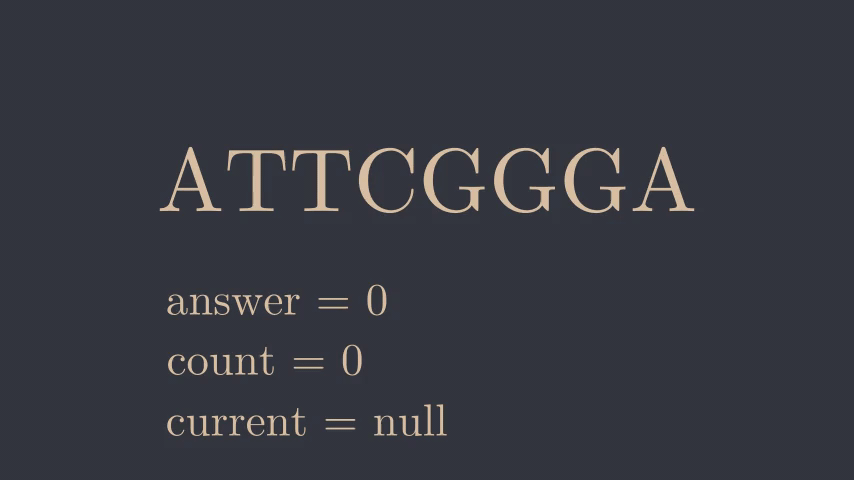# Repetitions Solution

3 September 2021

Statement

( The original statement can be found here )
You are given a DNA sequence: a string consisting of characters A, C, G, and T. Your task is to find the longest repetition in the sequence. This is a maximum-length substring containing only one type of character.

Input
The only input line contains a string of n characters.

Output
Print one integer: the length of the longest repetition.

Constraints
1 ≤ n ≤ $$10^6$$
Time limit : 1.00s
Memory limit : 512MB

Example

Input :
ATTCGGGA

Output :
3

Solution

We are given a DNA sequence and asked to find the longest contiguous segment containing the same character.

Since $$N$$ is at most $$10^6$$, there is a simple linear approach to this problem.

One possible solution is to iterate through the string and have a variable that stores the character of the current substring. If the character at the $$ith$$ iteration changes we update this variable, otherwise we increment the current count. At each iteration, we also update the answer to be $$max( answer, count)$$.

The following animation illustrates this algorithm using the sample input.#include <bits/stdc++.h>
using namespace std;

int main() {
string s;
char current;
int count = 0, answer = 0;
cin >> s;
for (int i = 0; i < s.length(); i++) {
if (s[i] != current) {
current = s[i];
count = 0;
}
if (s[i] == current) {
count++;
}

This solution has both time and space complexity of $$O(N)$$ where $$N$$ is the length of the string.